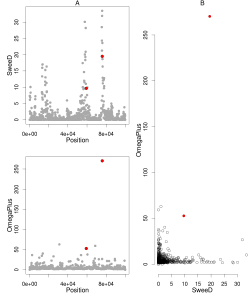# Combine OmegaPlus and SweeD – thresholds from simulations

Download the .tar.gz file that contains all necessary file for this demonstration.

combine_plots_simOutliers.tar.gz

I will demonstrate how to run the R script combine_plots_sims.R which combines the results of OmegaPlus with SweeD and detects the common outliers based on neutral simulations. Additionally, given a gff file with gene annotations it prints out the outlier genes.

Please use the latest versions of SweeD (>= 3.2.3) and OmegaPlus (>=2.2.4). These versions facilitate the import of the analysis results into R.

Please open and inspect the combine_plots_sims.R. Probably you will need to modify the file names.

The scirpt combine_plots_sims.R performs the following steps

1. performs neutral simulations. The user must set the variable “simulations” to 1 and also set the correct demographic model.
2. Based on the neutral simulations, it computes a threshold for the SweeD and for the OmegaPlus
3. It detects the points from a real (observation) dataset (not simulated), that are over the threshold
4. creates plots of OmegaPlus, SweeD and a combined plot
5. Given a gff file with the coordinates of genes on the genome, it finds out the outlier genes

To execute the script combine_plots_sims.R, you can either use the command line

` R CMD BATCH ./combine_plots_sims.R`

or open it with emacs+ess or rstudio to execute it line-by-line.

Results

The final result may look similar to the one below:In this figure, the left panel represents the score profile of SweeD and OmegaPlus; gray are the points where the score is calculated. Red are the common outliers based on neutral simulations. On the right panel you can see the combined plot OmegaPlus vs SweeD.